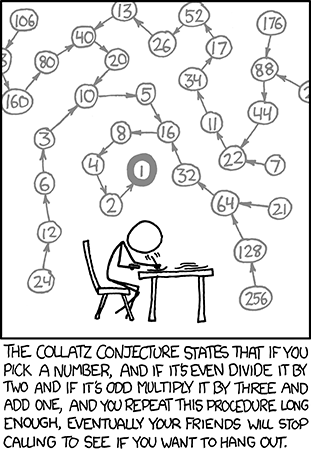# Maximum Path Sum — Dynamic Programming Algorithm

I came across this problem recently that required solving for the maximum-sum path in a triangle array.To copy the above triangle array:

As can be seen, there are 15 levels to this tree (including the top most node). Therefore, there are 214 possible routes to scan in order to check for the maximum sum using brute force. As there are only 214 (16384) routes, it is possible to solve this problem by trying every route. However, doing the same using brute force on a triangle array of 100 levels would take several billion years to solve using a computer that checks through say, 1012 routes per second. A greedy algorithm might per-chance work for the particular 4-level example problem stated above, but will not always work, and in most cases won’t. For instance, for the 100-level problem:

The Algorithm

Solving such a problem would require a powerful approach – and surely enough, there is an algorithm that solves the 100-level problem in a fraction of a second. Here’s a brief sketch of the algorithm:

You have such triangle:

``````   3
7 4
2 4 6
8 5 9 3
``````

Let’s say you’re on the penultimate level 2 4 6 and you have to iterate over it.

From 2, you can go to either 8 or 5, so 8 is better (maximize you result by 3) so you calculate the first sum 8 + 2 = 10

From 4, you can go to either 5 or 9, so 9 is better (maximize you result by 4) so you calculate the second sum 9 + 4 = 13

From 6, you can go to either 9 or 3, so 9 is better again (maximize you result by 6) so you calculate the third sum 9 + 6 = 15

This is the end of first iteration and you got the line of sums `10 13 15`.

Now you’ve got triangle of lower dimension:

``````      3
7    4
10   13    15
``````

Keep going this way…

``````         3
20    19
``````

…and you finally arrive at 23 as the answer.

The Code

Now for the Python code. I first store the 100-level triangle array in a text file, euler67.txt
I read the triangle array into Python and successively update the penultimate row and delete the last row according to the algorithm discussed above.

This code is the key to solving problems 18 and 67 of Project Euler.
Problem 18
Ans: 1074
Problem 67
Ans: 7273

# Collatz Conjecture — What You Need to Know

Like many of my previous posts, this post too has something to do with a Project Euler problem. Here’s a sketch of the Colatz Conjecture.

The following iterative sequence is defined for the set of positive integers:

n → n/2 (n is even)
n → 3n + 1 (n is odd)

Using the rule above and starting with 13, we generate the following sequence:

13 → 40 → 20 → 10 → 5 → 16 → 8 → 4 → 2 → 1

So basically, it’s just this. Take any natural number n. If n is even, divide it by 2 to get n / 2. If n is odd, multiply it by 3 and add 1 to obtain 3n + 1. Repeat the process indefinitely. The conjecture is that no matter what number you start with, you will always eventually reach 1. The property has aptly been called oneness! But perhaps oneness has its pitfalls too…If the conjecture is false, it can only be because there is some starting number which gives rise to a sequence that does not contain 1. Such a sequence might enter a repeating cycle that excludes 1, or increase without bound. No such sequence has been found.

Question
It can be seen that the sequence:
13 → 40 → 20 → 10 → 5 → 16 → 8 → 4 → 2 → 1
contains 10 terms. Although it has not been proved yet (Collatz Problem), it is thought that all starting numbers finish at 1. Which starting number, under one million, produces the longest chain?

NOTE: Once the chain starts the terms are allowed to go above one million.

HUGE HINT:

Histogram of stopping times for the numbers 1 to 100 million. Stopping time is on the x axis, frequency on the y axis.Approach 1 (A naïve, but straigh forward method)

Approach 2 (Smart, quick method that uses dynamic programming with the help of dictionaries)

I couldn’t help appreciate the elegance of the second algorithm. It’ll be well worth perusing if you don’t get it at one go. [Hint: It keeps track of the number of terms of a particular sequence as values assigned to keys of a Python dictionary]

Ans: 837799